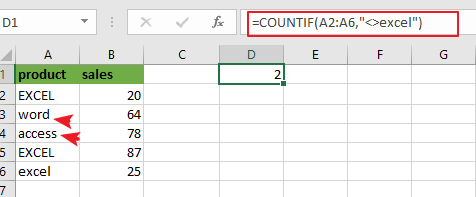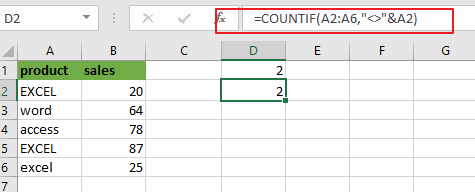# How to Count Cells Not Equal to a Specific Value in Excel

This post will guide you how to count the number of cells that are not equal to a specific value in a given range cells using a formula in Excel 2013/2016. Is there an easy way to count cells which are not equal to a particular value in your working data in Excel.

## Count Cells Not Equal to a Value

Assuming that you want to count cells that not contain value defined in a selected range of cells or count cells that are not equal to a specific value. In this case, Excel has provided a simple solution to count cells that are not equal to a certain value. And you can use the COUNTIF function to solve this problem very easily. The below steps will show you how to count cells not equal to another value in Excel.

To Count the number of cells in range A2:A6 that are not equal to “excel” without case sensitive, you can use the below formula based on the COUNTIF function:

=COUNTIF(A2:A6,”<>excel”)Let’s see how this formula works:

The COUNTIF function can be used to count the number of cells in a range that match the supplied criteria in Microsoft Excel. The range A2:A6 is the range of cells in which you want to match the criteria. The criteria “excel” is the value that you want to ignore during the count in the range “A2:A6”.

COUNTIF Function will count the number of cells in the range “`A2:A6`” that matches the condition you provide. And the “`not equal to operator`” will be used to count the cells in the range that does not equal to the value “excel”. And it will count all other values except this one.

The COUNTIF function is not case-sensitive. So if you wish to count cells exactly equal to a specific value, and you should use EXACT function.

Note: you can also use a value from a cell as its part of the criteria in the above COUNTIF function. To do this you need to use the ampersand operator to combine with that cell reference. Just like the below formula:

=COUNTIF(A2:A6,”<>”&A2)Note: the Cell A2 is the value that you want the cells not equal to in range A2:A6.

### Related Functions

• Excel COUNTIF function
The Excel COUNTIF function will count the number of cells in a range that meet a given criteria. This function can be used to count the different kinds of cells with number, date, text values, blank, non-blanks, or containing specific characters.etc.= COUNTIF (range, criteria)…
• Excel EXACT function
The Excel EXACT function compares if two text strings are the same and returns TRUE if they are the same, Or, it will return FALSE.The syntax of the EXACT function is as below:= EXACT (text1,text2)…